## Cipher## Mar 30, 2017

### Ivanka Trump is making her White House job official + Jared Kushner 666 Fifth avenueShe assumed office on 3/29/2017 which is exactly 151 days after her birthday
Ivanka Trump = 18+5+26+13+16+26 + 7+9+6+14+11 = 151 (Reverse Ordinal)Twenty ninth of March = 7+4+4+4+7+2 + 4+9+4+7+1 + 3+3 + 5+8+9+6+1 = 88 (Reverse Reduced)
Trump = 20+18+21+13+16 = 88 (Ordinal)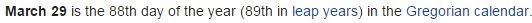It's also 216 days to her next birthday (6x6x6 = 216)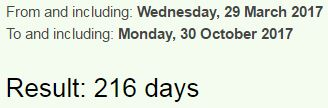She's born on 30th of October - Thirtieth of October = 20+8+9+18+20+9+5+20+8 + 15+6 + 15+3+20+15+2+5+18 = 216 (Ordinal)

http://edition.cnn.com/2017/03/29/politics/ivanka-trump-white-house-job/index.htmlIvanka Trump is making her White House job official = 9+4+1+5+2+1 + 2+9+3+4+7 + 9+1 + 4+1+2+9+5+7 + 8+5+9 + 5+8+9+2+5 + 8+6+3+1+5 + 1+6+2 + 6+6+6+9+3+9+1+3 = 211 (Reduced)
Ivanka Trump = 9+4+1+5+2+1 + 2+9+3+4+7 = 47 (Reduced)
Trump = 7+9+6+14+11 = 47 (Reverse Ordinal)She's known for being a boardroom judge on Trump's TV show "The Apprentice"
The Apprentice = 7+19+22 + 26+11+11+9+22+13+7+18+24+22 = 211 (Reverse Ordinal)
Judge = 10+21+4+7+5 = 47 (Ordinal)

She is "Assistant to the president"Thirty three = 7+19+18+9+7+2 + 7+19+9+22+22 = 141 (Reverse Ordinal)

On the day Ivanka took the white house job the media was talking about Jared Kushner -

It was a date with 42 numerology - 3/29/2017 - 3+29+2+0+1+7
"Kushner" = 2+3+10+8+5+5+9 = 42 (S Exception)"Anbang" = 8+4+7+8+4+2 = 33 (Reverse Reduced)
"Thirty three" = 2+8+9+9+2+7 + 2+8+9+5+5 = 66 (Reduced)
"Kushner company" = 2+3+1+8+5+5+9 + 3+6+4+7+1+5+7 = 66 (Reduced)
Ivanka married into Kushner family - "Kushner" = 2+3+1+8+5+5+9 = 33 (Reduced)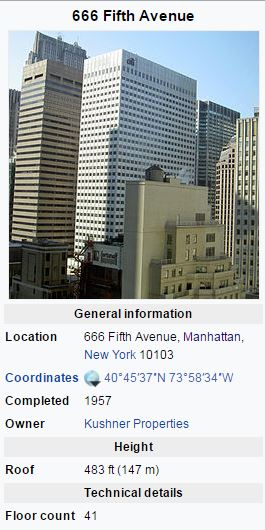Kushner properties = 2+3+1+8+5+5+9 + 7+9+6+7+5+9+2+9+5+1 = 93 (Reduced)
Kushner = 16+6+8+19+13+22+9 = 93 (Reverse Ordinal), Saturn = 93 (Ordinal)
Note that building is 147 meters tall (147 is 223 in octal system) both are very special numbers to Freemasonry

The building is 41 stories tall and this is coming exactly 41 weeks before Jared's next birthday (1/10/2018)## Mar 28, 2017

### CNN - 200-lb gold coin stolen from German museum (not really)

This has to be the most hilarious story of 2017 so far.. Absolutely nothing about this makes sense so I'll just ignore all the idiocy and just focus on the numbers, also the coin is definitely not stolen, this whole story is a joke... http://edition.cnn.com/2017/03/27/europe/german-coin-stolen-trnd/index.html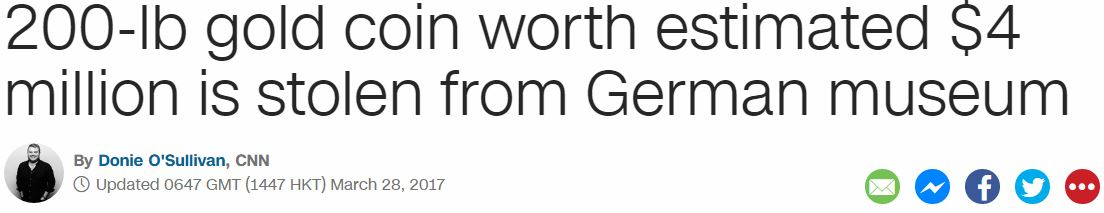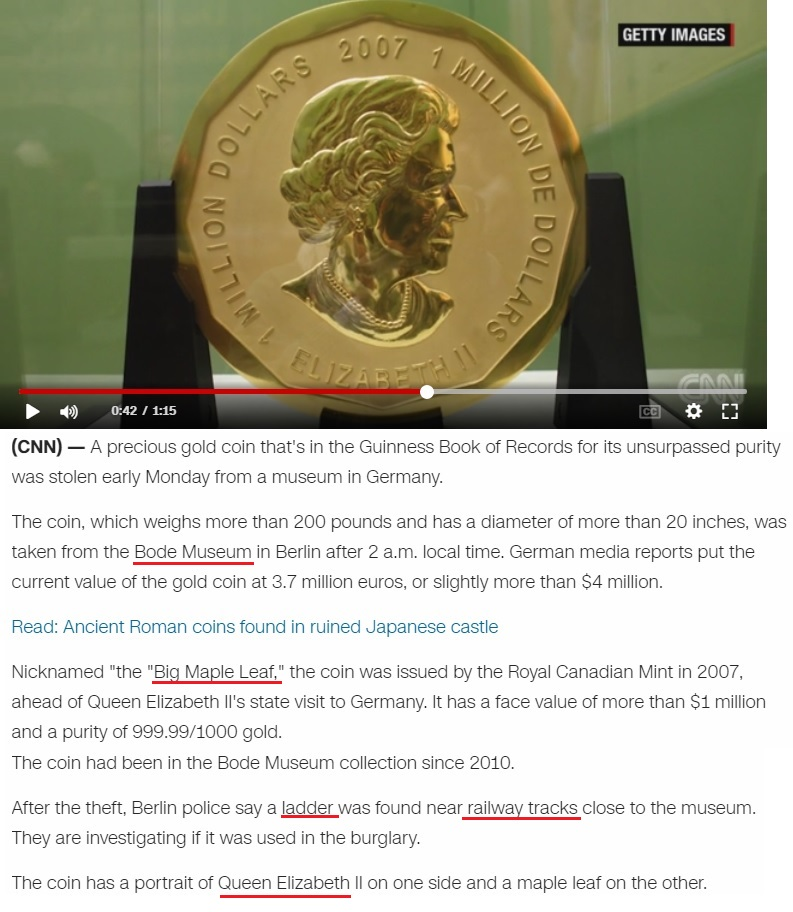This is coming on 87th day of the year - Twenty eighth of March = 7+4+4+4+7+2 + 4+9+2+1+7+1 + 3+3 + 5+8+9+6+1 = 87 (Reverse Reduced)
"Queen Elizabeth II" = 8+3+5+5+5 + 5+3+9+8+1+2+5+2+8 + 9+9 = 87 (Reduced)

Twenty eighth of March = 20+23+5+14+20+25 + 5+9+7+8+20+8 + 15+6 + 13+1+18+3+8 = 228 (Ordinal)
Queen Elizabeth = 10+6+22+22+13 + 22+15+18+1+26+25+22+7+19 = 228 (Reverse Ordinal
golden coin = 7+50+20+4+5+40 + 3+50+9+40 = 228 (Jewish)

The coin is nicknamed "Big Maple Leaf" = 2+9+7 + 4+1+7+3+5 + 3+5+1+6 = 53 (Reduced)
"golden coin" = 7+6+3+4+5+5 + 3+6+9+5 = 53 (Reduced)
It was sitting in "Bode Museum" = 7+3+5+4 + 5+6+8+4+6+5 = 53 (Reverse Reduced)
The coin has a face value of "one million" = 3+4+4 + 5+9+6+6+9+3+4 = 53 (Reverse Reduced)It's very important to note that coin is 53 centimeters in diameter - "Fifty three centimeters" = 21+18+21+7+2 + 7+19+9+22+22 + 24+22+13+7+18+14+22+7+22+9+8 = 314 (Reverse Ordinal)The ladder was supposedly found near "railway tracks" = 9+1+9+3+5+1+7 + 2+9+1+3+2+1 = 53 (Reduced)

Bode Museum is located in Berlin, Germany - https://en.wikipedia.org/wiki/Bode_MuseumThis is coming on 28th day of the month (33 and 28 are the only 2 numbers that sum to 156 in the first 300)
"Twenty eight" = 20+23+5+14+20+25 + 5+9+7+8+20 = 156 (Ordinal)

German Museum = 2+4+9+5+8+4 + 5+6+8+4+6+5 = 66 (Reverse Reduced)
Queen Elizabeth = 1+6+4+4+4 + 4+6+9+1+8+7+4+7+1 = 66 (Reverse
On Wikipedia they call it a  "solid gold coin" = 1+6+3+9+4 + 7+6+3+4 + 3+6+9+5 = 66 (Reduced)
This is happening 24 days before Elizabeth's birthday which is also 3 weeks and 3 days (note that coin is 24-carat gold)

This happened on a fitting date numerology

3/28/2017 = 3+2+8+2+0+1+7 = 23 - "Coin" = 3+6+9+5 = 23 (Reduced)
3/28/2017 = 3+28+2+0+1+7 = 41 - "Burglary" = 2+3+9+7+3+1+9+7 = 41 (Reduced), "Coin" = 3+15+9+14 = 41 (Ordinal)

There are also some 118's popping up
"Ladder" = 15+26+23+23+22+9 = 118 (Reverse Ordinal)
"fifty three centimeters" = 6+9+6+2+7 + 2+8+9+5+5 + 3+5+5+2+9+4+5+2+5+9+10 = 118 (S Exception)
"one million" = 15+14+5 + 13+9+12+12+9+15+14 = 118 (Ordinal)
"Bode Museum" = 2+15+4+5 + 13+21+19+5+21+13 = 118 (Ordinal)
Bode Museum was reopened on 10/18/2006

## Mar 24, 2017

### CNN - New kind of cloud - Are we going to start seeing more of these?

Story - http://edition.cnn.com/2017/03/23/us/asperitas-added-international-cloud-atlas-trnd/index.html

Something tells me that we are gonna start seeing more of these clouds being produced in the future...  they are surely not stopping with weather modification any time soon..

These are some of the pictures from their postLook! Up in the sky! Its a new kind of cloud = 30+60+60+20 + 300+70 + 9+50 + 200+8+5 + 100+20+700 + 9+200+100 + 1 + 50+5+500 + 20+9+50+4 + 60+6 + 3+30+60+300+4 = 3043 (English)
7x7x7 = 343 (227 is 343 in octal numbering system)
The story is released on "March Twenty fourth" = 30+1+80+3+8 + 100+900+5+40+100+400 + 6+50+200+80+100+8 = 2111 (Jewish)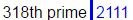7/22 = 0.318
22/7 = 3.14

"The World Meteorological Organization had not updated the atlas in 30 years, until now."

Astrologers usually round out Saturn's orbit at 30 years, lately I've started noticing more and more how they love to do things 30 years apart
three decades = 7+19+9+22+22 + 23+22+24+26+23+22+8 = 227 (Reverse Ordinal)
"The cube of Saturn" = 7+19+22 + 24+6+25+22 + 12+21 + 8+26+7+6+9+13 = 227 (Reverse Ordinal)They are calling it "Asperitas cloud" = 1+1+7+5+9+9+2+1+1 + 3+3+6+3+4 = 55 (Reduced)
"cloud" = 3+12+15+21+4 = 55 (Ordinal)
"sky" = 19+11+25 = 55 (Ordinal)
"heaven" = 8+5+1+22+5+14 = 55 (Ordinal)

Note that World Meteorological day comes on 3/23, a day after 3/22, the number of Skull & Bones
The cloud was officially recognized on 3/23 but CNN is reporting it later for coding numerological reasons
"March Twenty Third" = 14+26+9+24+19 + 7+4+22+13+7+2 + 7+19+18+9+23 = 223 (Reverse Ordinal)
"Asperitas clouds" = 26+8+11+22+9+18+7+26+8 + 24+15+12+6+23+8 = 223 (Reverse Ordinal)
"Two two three" = 2+5+6 + 2+5+6 + 2+8+9+5+5 = 55 (Reduced)

## Mar 23, 2017

### Bill Hicks = Alex Jones - Beautiful gematria script

There's a lot of people showing evidence that Bill Hicks is Alex Jones but when we look at gematria we see insane relationship between these two charactersBill Hicks "died" on 2/26/1994 - 2+2+6+1+9+9+4 = 33 - he was born in "Valdosta Georgia" = 4+1+3+4+6+1+2+1 + 7+5+6+9+7+9+1 = 66 (Reduced)
"Valdosta Georgia" = 22+1+12+4+15+19+20+1 + 7+5+15+18+7+9+1 = 156 (Ordinal)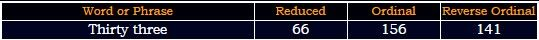2/26/1994 - 2+26+19+94 = 141
He's born on 16th day of the December - Sixteen = 1+9+6+2+5+5+5 = 33 (Reduced)

Note that Alex Jones is supposedly born in "Dallas, Texas" = 4+1+3+3+1+1 + 2+5+6+1+1 = 28 (Reduced)
Hicks was born on 12/16 = 28 and he died on 2/26 which also sums to 28..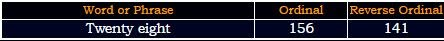156th prime number is 911
Note that Alex Jones is probably most known for his 9/11 stuff and Bill Hicks died EXACTLY 1 year after 1993 World Trade Center Bombing
"one year" = 60+50+5 + 700+5+1+90 = 911 (English)
Bill Hicks is listed as a musician and comedian, he "died" at Little Rock which connects to his profession
"Little Rock" = 3+9+2+2+3+5 + 9+6+3+2 = 44 (Reduced)
comedian = 6+3+5+4+5+9+8+4 = 44 (Reverse Reduced)
musician = 4+3+10+9+3+9+1+5 = 44 (S Exception)
He was also born in "December" = 5+4+6+4+5+7+4+9 = 44 (Reverse Reduced)
He supposedly died from "Pancreatic cancer"
Pancreatic cancer = 7+1+5+3+9+5+1+2+9+3 + 3+1+5+3+5+9 = 71 (Reduced)
cancer = 3+1+14+3+5+18 = 44 (Ordinal)Magnolia = 14+26+20+13+12+15+18+26 = 144 (Reverse Ordinal)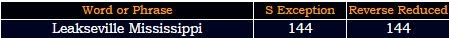Hicks was born in "Georgia" = 7+5+6+9+7+9+1 = 44 (Reduced)

Now here is the best part
"Bill Hicks" = 2+9+30+30 + 8+9+3+20+100 = 211 (English)
He died 2 months and 11 days after his birthdayAlex Jones is born on 2/11 - the 42nd day of the year
"February" = 6+5+2+9+3+1+9+7 = 42 (Reduced)
Forty second day = 6+6+9+2+7 + 1+5+3+6+5+4 + 4+1+7 = 66 (Reduced)
Alex Jones = 1+3+5+6 + 1+6+5+5+1 = 33 (Reduced)
Alex Jones = 1+3+5+6 + 1+6+5+5+10 = 42 (S Exception)
InfoWars = 9+5+6+6+5+1+9+1 = 42 (Reduced)

His last comedy special is called Revelations and it was aired on September 14th, 93 days before his birthdayHis birthday in 1993 landed on a date with 32 numerology (12/16/1993 - 1+2+1+6+1+9+9+3 = 32)
Bill Hicks died at the age of 32 - "Hicks" = 8+9+3+2+10 = 32 (S Exception)
World Trade Center bombing took place on  2/26/93 "Two hundred twenty six" = 2+5+6 + 8+3+5+4+9+5+4 + 2+5+5+5+2+7 + 1+9+6 = 93 (Reduced)

Check out this video, this guy shows pretty nice proof of them being the same person
https://www.youtube.com/watch?v=GjfIts2CGbA&t=340s

## Mar 21, 2017

### CNN - 33 incidents in 2017 - 33/Pi tributes on a 33 date numerology

CNN's story -Today is March 20th, a date with 33 numerology and CNN released a story giving a lot of tributes to 33, there is also some interesting Pi coding in this article starting from the time of release 22:07

The first sentence has 227 gematria of course
A mosque in Ypsilanti Michigan is burned to the ground = 1 + 4+6+1+8+3+5 + 9+5 + 7+7+1+9+3+1+5+2+9 + 4+9+3+8+9+7+1+5 + 9+1 + 2+3+9+5+5+4 + 2+6 + 2+8+5 + 7+9+6+3+5+4 = 227 (Reduced)2nd sentence sums to 364, much like a full year/full circleRoseville California = 9+6+1+5+4+9+3+3+5 + 3+1+3+9+6+6+9+5+9+1 = 97 (Reduced)
25th prime number is 97 (Pi = 25)
threats, vandalism or arson = 2+8+9+5+1+2+1 + 4+1+5+4+1+3+9+1+4 + 6+9 + 1+9+1+6+5 = 97 (Reduced)
incidents = 9+14+3+9+4+5+14+20+19 = 97 (Ordinal)
March = 4+1+9+3+8 = 25 (Reduced)
Pi = 16+9 = 25 (Ordinal)

Council on American-Islamic Relations = 3+6+3+5+3+9+3+6+5+1+4+5+9+9+3+1+5+9+1+3+1+4+9+3+9+5+3+1+2+9+6+5+1 = 151 (Reduced)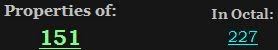Florida to Hawaii = 6+12+15+18+9+4+1 + 20+15 + 8+1+23+1+9+9 = 151 (Ordinal)

3/20/2017 - 3+20+2+0+1+7 = 33
They already made sure to highlight certain things for us, like 33 incidents from Janaury 1st to March 20th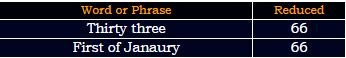There is 79 days from January 1st to the release of this article (22nd prime number is 79), also it is 2 months and 20 days a lot like 22 again, which connects to the circle and 22/7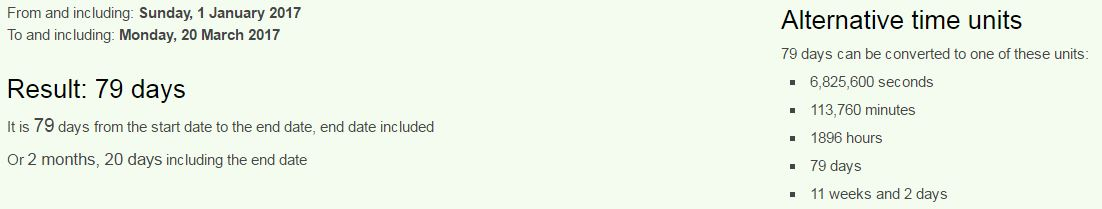It is also 11 weeks and 2 days - Circle = 24+18+9+24+15+22 = 112 (Reverse Ordinal)

They say that in 2016 there were 17 incidents in the same time period
33-17 = 16 - Sixteen = 1+9+6+2+5+5+5 = 33 (Reduced)
Pi = 7+9 = 16 (Reduced)

The incidents, tallied by the Council on American-Islamic Relations, span 19 states from Florida to Hawaii.

Hawaii = 8+1+5+1+9+9 = 33 (Reduced)Admission to union on 3/3? 27 is 33 in octal system as well

Below the text they have listed 33 places where those "incidents" happened and the last one on the list just happens to sum to 777
Bellevue = 2+5+30+30+5+400+300+5 = 777 (English)

## Mar 19, 2017

### Syrian city of Homs evacuation - 3/18/2017 - 314/318/227 coding

CNN's post - http://edition.cnn.com/2017/03/18/middleeast/syria-conflict-rebels-evacuated/index.html

I love catching smaller articles like this because the coding is always really easy to pick upIn Syrian city of Homs an orderly evacuation of rebels = 9+5 + 1+7+9+9+1+5 + 3+9+2+7 + 6+6 + 8+6+4+1 + 1+5 + 6+9+4+5+9+3+7 + 5+4+1+3+3+1+2+9+6+5 + 6+6 + 9+5+2+5+3+1 = 223 (Reduced)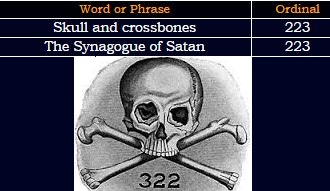This story is coming on March 18th - written 3/18, and connecting to the first line of this story, the fact that story is coming on this date means that it will have circle and Saturn codingAlso we find 318 in story highlights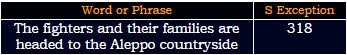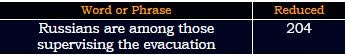318 is a number connecting to Pi
7/22 = 0.318
22/7 = 3.14

The state-run Syrian Arab News Agency is reporting that the first batch of "gunmen and their families" are leaving the city's Al-Waer neighborhood.Syrian Arab News Agency = 10+7+9+9+1+5 + 1+9+1+2 + 5+5+5+10 + 1+7+5+5+3+7 = 107 (S Exception)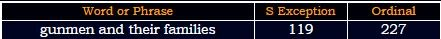119+191+911 = 1221 - 12/21 is the first day of Capricorn (Ruled by Saturn) - Saturn = 119 (Francis Bacon)Star of David = 119 (Ordinal)All seeing eye = 119 (Ordinal)
227+272+722 = 1221
Russian supervision = 9+6+8+8+9+8+4 + 8+6+2+4+9+5+9+8+9+3+4 = 119 (Reverse Reduced)
"Evacuation" = 5+700+1+3+200+1+100+9+50+40 = 1109 (Jewish)
The Homs Media Center, an activist group, is reporting that 2,000 residents including 400 rebel fighters have left Al-Waer and are heading to Jarablus in the Aleppo countryside.
"Al-Waer" = 27+12+49+1+5+18 = 112 (Francis Bacon)
"Circle" = 24+18+9+24+15+22 = 112 (Reverse Ordinal)Also look at the coordinates - 36° N, 38° E - Syria = 8+2+9+9+8 = 36 (Reverse Reduced)
3/18/17 - 3+18+17 = 38

People are moving from "city of Homs" = 24+18+7+2 + 12+21 + 19+12+14+8 = 137 (Reverse Ordinal) - 137 is 33rd prime number
to "Jarabulus" = 1+1+9+1+2+3+3+3+10 = 33 (S Exception)
Also 2000 residents are heading to Jarabulus
"two thousand" = 7+4+12 + 7+19+12+6+8+26+13+23 = 137 (Reverse Ordinal)
Weird.. right? I think there's no reason to decode this story any further, it's clear why all these numbers appeared

## Mar 15, 2017

### CNN - Maria Sharapova suspension, winning Wimbledon 2017, incredible coding

CNN's story -

A few months ago I saw a story on Sharapova and had some pretty interesting finds in relation to her "drug ban" so I decided to wait for the next story which is the one coming today on March 15thNote the length of the video - 3:43 (7x7x7) , she failed a drug test on 26th of January - 3:43 is 223 seconds (The Synagogue of Satan = 223, the number of Skull and Bones is 322)
Twenty sixth of January = 100+900+5+40+100+400 + 90+9+300+100+8 + 50+6 + 600+1+40+200+1+80+400 = 3430 (Jewish)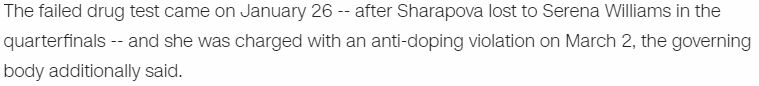1/26/16 = 1+26+16 = 43 (The news of the failed test came 42 days later on 3/7/2016 -  "Maria" = 13+1+18+9+1 = 42 (Ordinal), "Sharapova" = 8+19+26+9+26+11+12+5+26 = 142 (Reverse Ordinal)"Maria Sharapova" = 1+1+5+5+1 + 6+6+1+5+1+3+2+5+1 = 43 (Septenary)
She tested positive for "Meldonium" = 4+5+3+4+6+5+9+3+4 = 43 (Reduced)
The mainstream media started covering story about failed test on March 7th, which was also 43 days before her next birthday
"March" = 13+1+18+3+8 = 43 (Ordinal)She's born on 19th of April
Nineteenth of April = 14+9+14+5+20+5+5+14+20+8 + 15+6 + 1+16+18+9+12 = 191 (Ordinal)Her full name is "Maria Yuryevna Sharapova" = 4+1+9+9+1 + 7+3+9+7+5+4+5+1 + 10+8+1+9+1+7+6+4+1 = 112 (S Exception)"Sharapova eligible to return on April 26" = 45+8+1+18+1+16+15+22+1 + 5+12+9+7+9+2+12+5+20+15+18+5+20+21+18+14+15+14+27+16+18+9+12 = 430 (Francis Bacon)
"Twenty sixth of April" = 2+5+5+5+2+7 + 1+9+6+2+8 + 6+6 + 1+7+9+9+3 = 93 (Reduced)
"Maria" = 14+26+9+18+26 = 93 (Reverse Ordinal)
From the release of this story there are 43 days to April 26th
April = 8+2+9+9+6 = 34 (Reverse Reduced)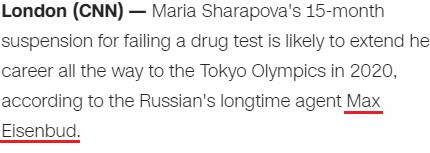Max = 14+26+3 = 43 (Reverse Ordinal)
Eisenbud = 5+9+10+5+5+2+3+4 = 43 (S Exception)
Maria Sharapova = 13+1+18+9+1 + 19+8+1+18+1+16+15+22+1 = 143 (Ordinal)
On the wikipedia we find that she has lost 43 games in Grand Slam TournamentsHer name and birthday have the same gematria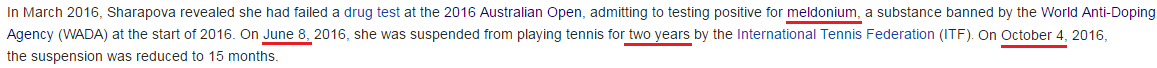"Two year ban" = 2+5+6 + 7+5+1+9 + 2+1+5 = 43 (Reduced)
She was suspended on 6/8 and then on 10/4 they decided to reduce the ban to 15 months
6+8 = 14 (6+8+20+16 = 50)
10+4 = 14 , both of these dates have date numerology of 50 (10+4+20+16) - "Circle" = 3+9+18+3+12+5 = 50 (Ordinal), America = 1+13+5+18+9+3+1 = 50 (Ordinal)
43 is the 14th prime number
Maria made her professional tennis debut in 2001 at the age of 14
In 2017 it's going to be her 14th time on Wimbledon (43)

From 6/8/2016 to 10/4/2016 is 119 days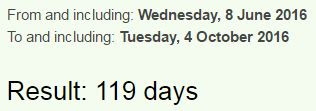She was suspended 135 days after failing a drug test (135+531 = 666) - Every angle in an octagon has 135° - one hundred thirty five = 60+50+5 + 8+300+50+4+90+5+4 + 200+8+9+90+200+700 + 6+9+400+5 = 2203 (English)From March 7th release of failed test to her suspension is 93 daysThere's definitely many connections with Saturnian numbers here
Saturn = 19+1+20+21+18+14 = 93 (Ordinal)
Saturn = 45+1+20+21+18+14 = 119 (Francis Bacon)
Star of David = 19+20+1+18 + 15+6 + 4+1+22+9+4 = 119 (Ordinal)
All Seeing eye = 1+12+12 + 19+5+5+9+14+7 + 5+25+5 = 119 (Ordinal)...

Twenty twenty Tokyo Olympics = 7+4+4+4+7+2 + 7+4+4+4+7+2 + 7+3+7+2+3 + 3+6+2+5+2+9+6+8 = 119 (Reverse Reduced)

The last tournament she played was "Australian Open" = 1+3+10+2+9+1+3+9+1+5 + 6+7+5+5 = 67 (S Exception)
Information about her failed test was released on 67th day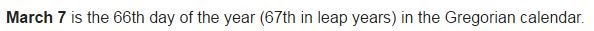sixty seventh day = 1+9+6+2+7 + 1+5+4+5+5+2+8 + 4+1+7 = 67 (Reduced)
"Seventh of March" = 1+5+4+5+5+2+8 + 6+6 + 4+1+9+3+8 = 67 (Reduced)
Her ban was shortened on "October Fourth" = 6+3+2+6+2+5+9 + 6+6+3+9+2+8 = 67 (Reduced)Stuttgart, Germany = 1+2+3+2+2+7+1+9+2 + 7+5+9+4+1+5+7 = 67 (Reduced)
April 26 is a date with 67 numerology (4+26+20+17)

From March 7th to October 4th is 211 days (47th prime number)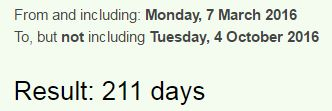33 and 47 are the numbers that often go together and their primes have a relationship through octal system, also there's a lot of 112 coding with this story, the reflection of 211
"Wimbledon" = 4+9+5+7+6+4+5+3+4 = 47 (Reverse Reduced)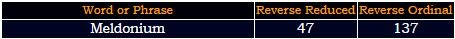This year Wimbledon is starting on 7/3 with a date numerology of 47 (7+3+20+17) and it ends on a date with 33 numerology 7/16/2017 (7+16+2+0+1+7)

From the suspension (6/8/2016) to her game in April is 322 days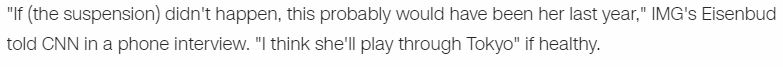Notice how in the next part of this story they are saying how she is healthy and recovered from the injuries and now her career is going to be extended because of the rest she got from the suspension. I think the entire reason they put Meldonium on the list at the start of 2016 is to have her suspended in order to have her play for a few more years... and based on this coding I would say she is going to win both Wimbledon 2017 and Tokyo Olympics. In regards to Meldonium, some experts say that there is no evidence that indicates it is a performance enhancer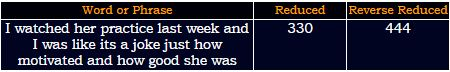Wimbledon = 5+9+4+2+3+5+4+6+5 = 43 (Reduced)
Wimbledon is starting on July 3rd and it ends on the 16th (Sixteen = 33 - Ordinal)
Sixteenth = 1+9+6+2+5+5+5+2+8 = 43 (Reduced)
Her birthday this year is landing on a date with 33 numerology (4+19+2+0+1+7) and the Wimbledon is ending on a date that has exactly the same date numerology as her birthdayJuly Sixteenth = 1+3+3+7 + 10+9+6+2+5+5+5+2+8 = 66 (S Exception)66 decimal places of Pi sum to 322
156th Fibonacci number has 33 digits - 178890334785183168257455287891792

She failed a drug test when she was "Twenty eight" = 20+23+5+14+20+25 + 5+9+7+8+20 = 156 (Ordinal)
Also she was banned by "International Tennis Federation"Thirty three = 7+19+18+9+7+2 + 7+19+9+22+22 = 141 (Reverse Ordinal)
Her first game after suspension is at Porsche Tennis Grand Prix tournament in Stuttgart
Porsche Tennis Grand Prix = 7+6+9+10+3+8+5 + 2+5+5+5+9+10 + 7+9+1+5+4 + 7+9+9+6 = 141 (S Exception)

The tournament is starting on 4/22 and it ends on 4/30April 22nd has date numerology of 43 like connecting to everything mentioned above
4/22/17 - 4+22+17 = 43 , she failed a drug test on 1/26/16 = 1+26+16 = 43
Tournament ends on 4/30 = 34
If she wins (and she probably will) it will be her fourth title on Stuttgart tournament
"fourth title" = 21+12+6+9+7+19 + 7+18+7+15+22 = 143 (Reverse Ordinal)
"Maria Sharapova" = 13+1+18+9+1 + 19+8+1+18+1+16+15+22+1 = 143 (Ordinal)

Porsche Tennis Grand Prix = 11+12+9+8+24+19+22 + 7+22+13+13+18+8 + 20+9+26+13+23 + 11+9+18+3 = 318 (Reverse Ordinal)
7/22 = 0.318

There are 2 months and 27 days from her birthday to the end of Wimbledon 2017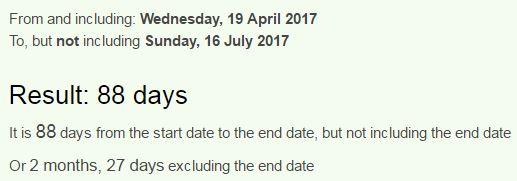22/7 = 3.14
From 3/15/2017 to her birthday is 1 month and 12 days a lot like 112
"Circle" = 24+18+9+24+15+22 = 112 (Reverse Ordinal)
"Olympics" = 15+12+25+13+16+9+3+19 = 112 (Ordinal)
"Tokyo" = 46+15+11+25+15 = 112 (Francis Bacon)
The Mainstream media started covering her story 112 days before the start of Wimbledon 2016It's very interesting how they started talking about her on 3/7 and Wimbledon in 2017 is coming on 7/3...
This would be her "sixth championship" = 112 (S Exception) title
"Maria Yuryevna Sharapova" = 4+1+9+9+1 + 7+3+9+7+5+4+5+1 + 10+8+1+9+1+7+6+4+1 = 112 (S Exception)

Think about how Maria Sharapova = 143 (Ordinal), missed Rio Olympics = 143 (Reverse Ordinal) last year
The rest of 2017 might be the best year of her career, her alignment with the tournaments is completely ridiculous